## 课程设计要求## 源码

myos2.c

#include<linux/init.h>
#include<linux/module.h>
#include<linux/kernel.h>
#include <linux/module.h>
#include <linux/fs.h>
#include <linux/string.h>
#include <asm/uaccess.h>
#include <linux/slab.h>
static int hello_init(void){
int maxA={0};
int maxB={0};
int maxC={0};
char *pa,*pb,*pc;
pa=(char*)kmalloc(sizeof(char)*100,GFP_KERNEL);
pb=(char*)kmalloc(sizeof(char)*100,GFP_KERNEL);
pc=(char*)kmalloc(sizeof(char)*100,GFP_KERNEL);
struct file *fp_a;
struct file *fp_b;
struct file *fp_c;
mm_segment_t fs;
loff_t pos;
fp_a = filp_open("a.txt", O_RDWR, 0644);
fp_b = filp_open("b.txt", O_RDWR, 0644);
fp_c = filp_open("c.txt",O_WRONLY | O_APPEND, 0644);

if (IS_ERR(fp_a)) {
printk("open file a.txt error\n");
return -1;
}
if (IS_ERR(fp_b)) {
printk("open file b.txt error\n");
return -1;
}
if (IS_ERR(fp_c)) {
printk("open file c.txt error\n");
return -1;
}
fs = get_fs();
set_fs(KERNEL_DS);
pos = 0;
pos=0;

sscanf(pa,"%d %d %d\n%d %d %d\n%d %d %d",&maxA,&maxA,&maxA,&maxA,&maxA,&maxA,&maxA,&maxA,&maxA);

sscanf(pb,"%d %d %d\n%d %d %d\n%d %d %d",&maxB,&maxB,&maxB,&maxB,&maxB,&maxB,&maxB,&maxB,&maxB);

int i;
for(i=0;i<3;i++){
int j;
for(j=0;j<3;j++){
int k;
for(k=0;k<3;k++){
maxC[i][j]=maxC[i][j]+maxA[i][k]*maxB[k][j];
}
}
}

sprintf(pc,"%d %d %d\n%d %d %d\n%d %d %d\n",maxC,maxC,maxC,maxC,maxC,maxC,maxC,maxC,maxC);

int size=0;
int m;
for(m=0;m<3;m++){
int n;
for(n=0;n<3;n++){
int tsize=0;
while(maxC[m][n]>0)
{
tsize++;
maxC[m][n]/=10;
}
size+=tsize+1;
}
}
pos=0;
kernel_write(fp_c,pc,size,&pos);
filp_close(fp_a, NULL);
filp_close(fp_b, NULL);
filp_close(fp_c, NULL);
set_fs(fs);

return 0;
}
static void hello_exit(void){
}
module_init(hello_init);
module_exit(hello_exit);
MODULE_LICENSE("GPL");

Makefile

obj-m :=os2.o
os2-objs:=myos2.o
KDIR :=/usr/src/linux-5.6.2
PWD :=$(shell pwd) default: make -C$(KDIR) M=$(PWD) modules clean: make -C$(KDIR) M=\$(PWD) clean

## 主要函数及解释说明

• set_fs()/get_fs():

• filp_open():

• filp_close():

• kmalloc()/kfree():

  ssize_t vfs_read(struct file *file, char __user *buf, size_t count, loff_t *pos)
参数中__user 表示buf是用户态指针，在内核中无法使用，因此需要设置使用环境，如下：
mm_segment_t old_fs;
old_fs = get_fs();
set_fs(get_ds());
set_fs(old_fs);

• vfs_write():

• sprintf():

sprintf指的是字符串格式化命令，函数声明为 int sprintf(char string, char format [,argument,...]);，主要功能是把格式化的数据写入某个字符串中，即发送格式化输出到 string 所指向的字符串。sprintf 是个变参函数。使用sprintf 对于写入buffer的字符数是没有限制的，这就存在了buffer溢出的可能性。解决这个问题，可以考虑使用 snprintf函数，该函数可对写入字符数做出限制。

• sscanf():

sscanf 读取格式化的字符串中的数据。原型：int sscanf(const char buffer,const char format,[argument] ... );

buffer 存储的数据

format 窗体控件字符串。

argument 可选自变量

locale 要使用的区域设置

## 实现过程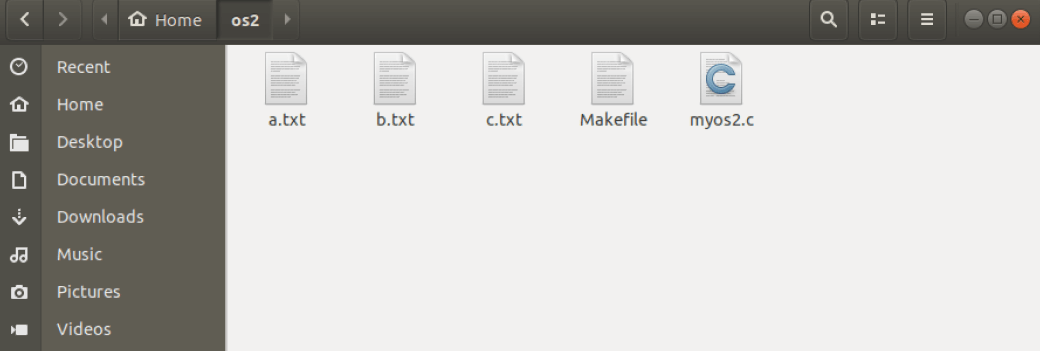a.txt,b.txt中内容均为下（c.txt为空）

1 2 3
4 5 6
7 8 9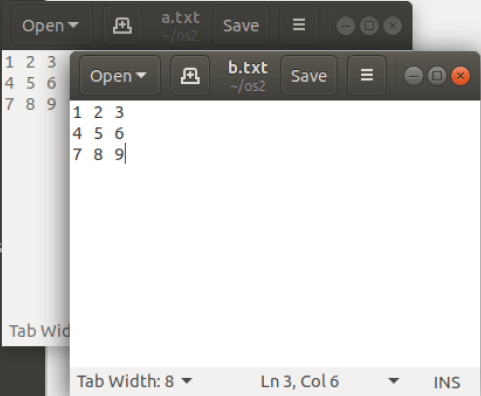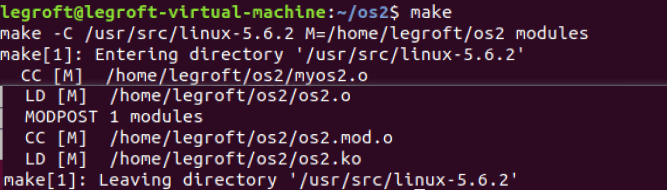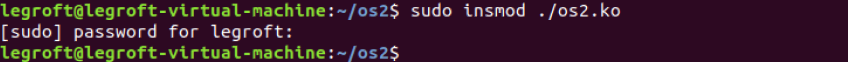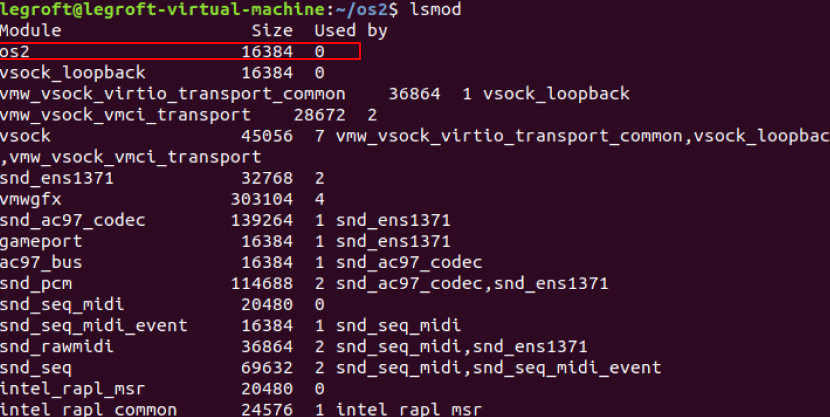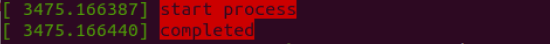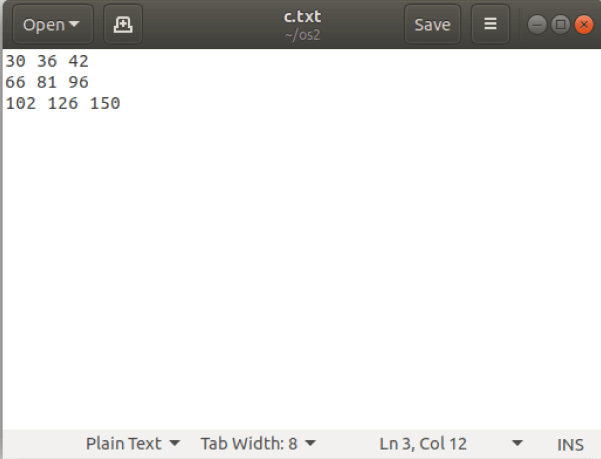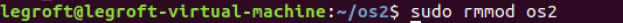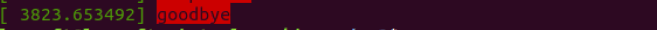Last modification：July 12th, 2020 at 01:03 am